# Lectures

The accompanying material for each lecture is posted here.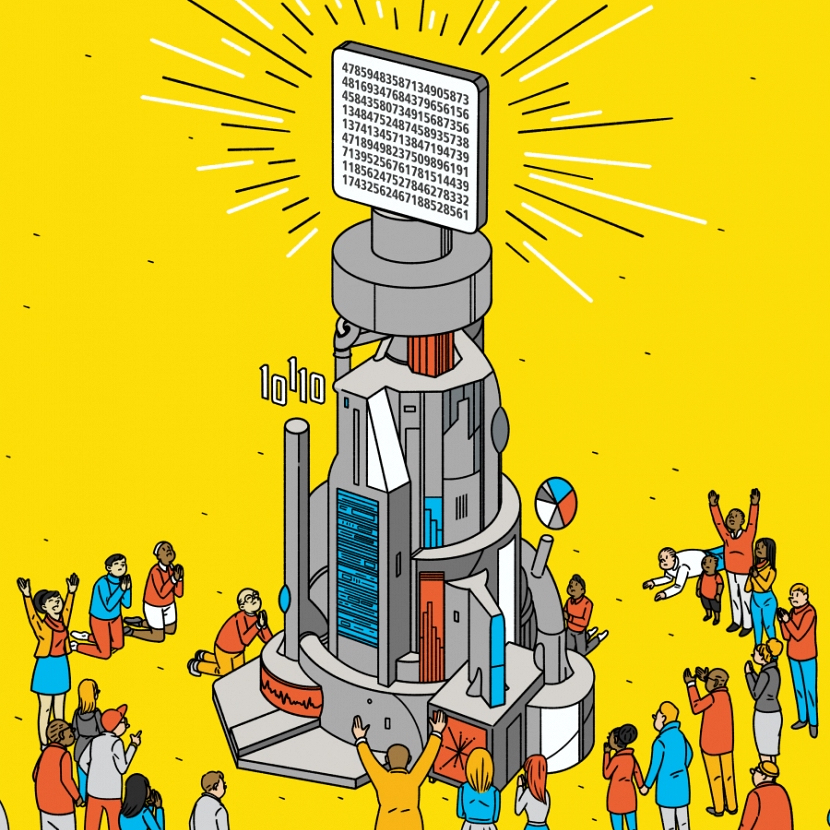## Lecture 1: Introduction

Origins of deep learning, course goals, overview of machine-learning paradigms, intro to computational acceleration.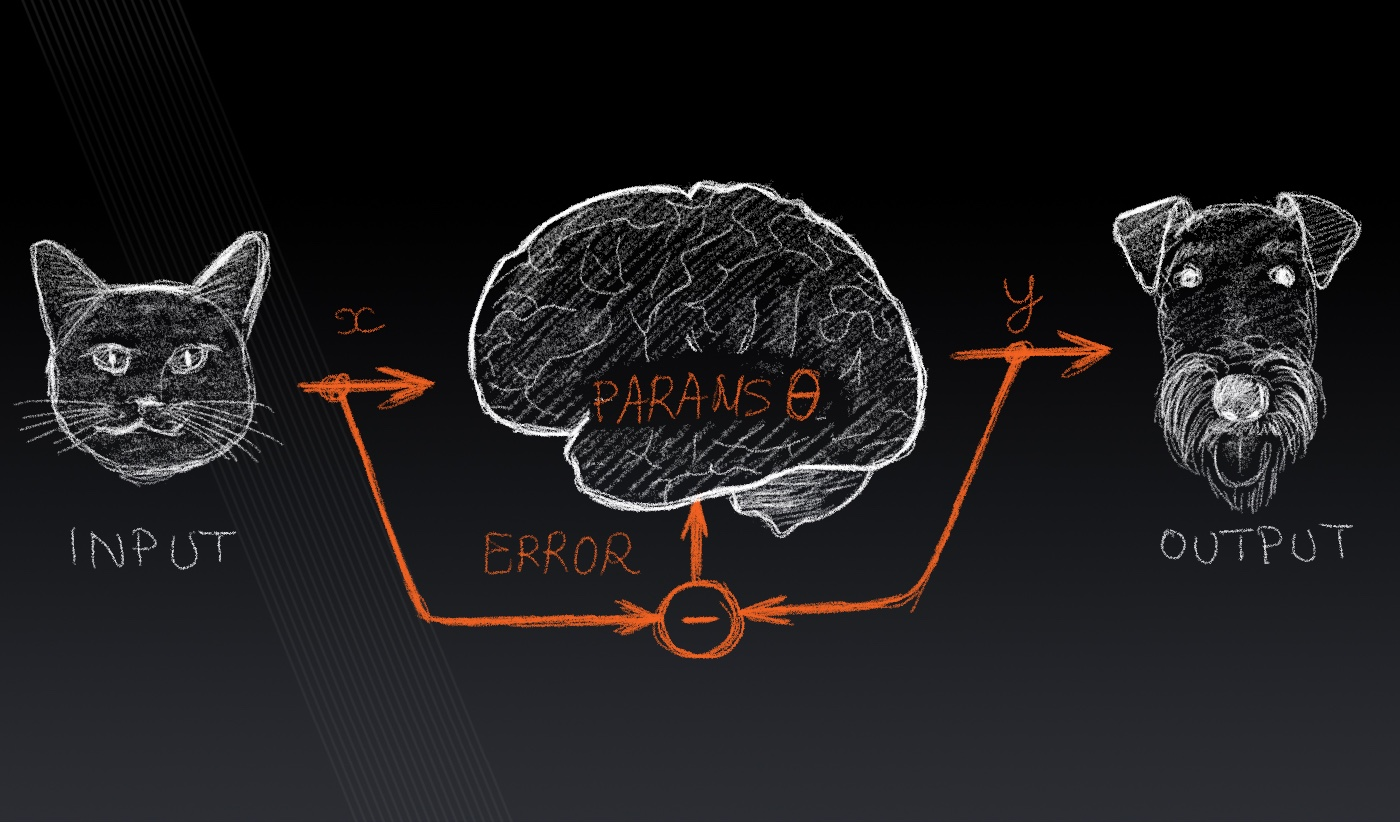## Lecture 2: Supervised learning

Supervised learning problem statement, data sets, hypothesis classes, loss functions, basic examples of supervised machine learning models, adding non-linear...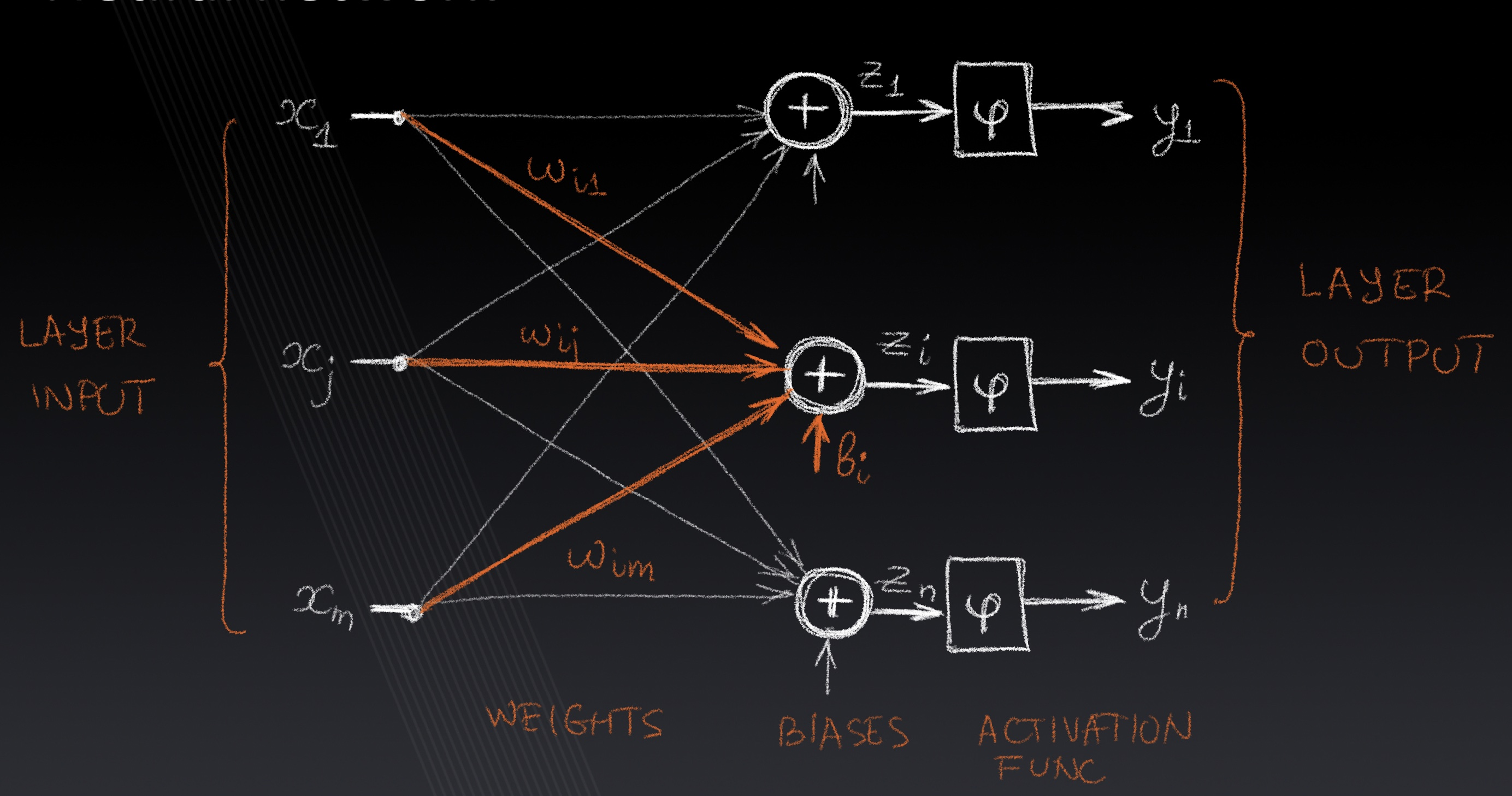## Lecture 3: Neural Networks

Linear and multilayer Perceptron, loss functions, activation functions, pooling, weight sharing, convolutional layers, gradient descent, backpropagation.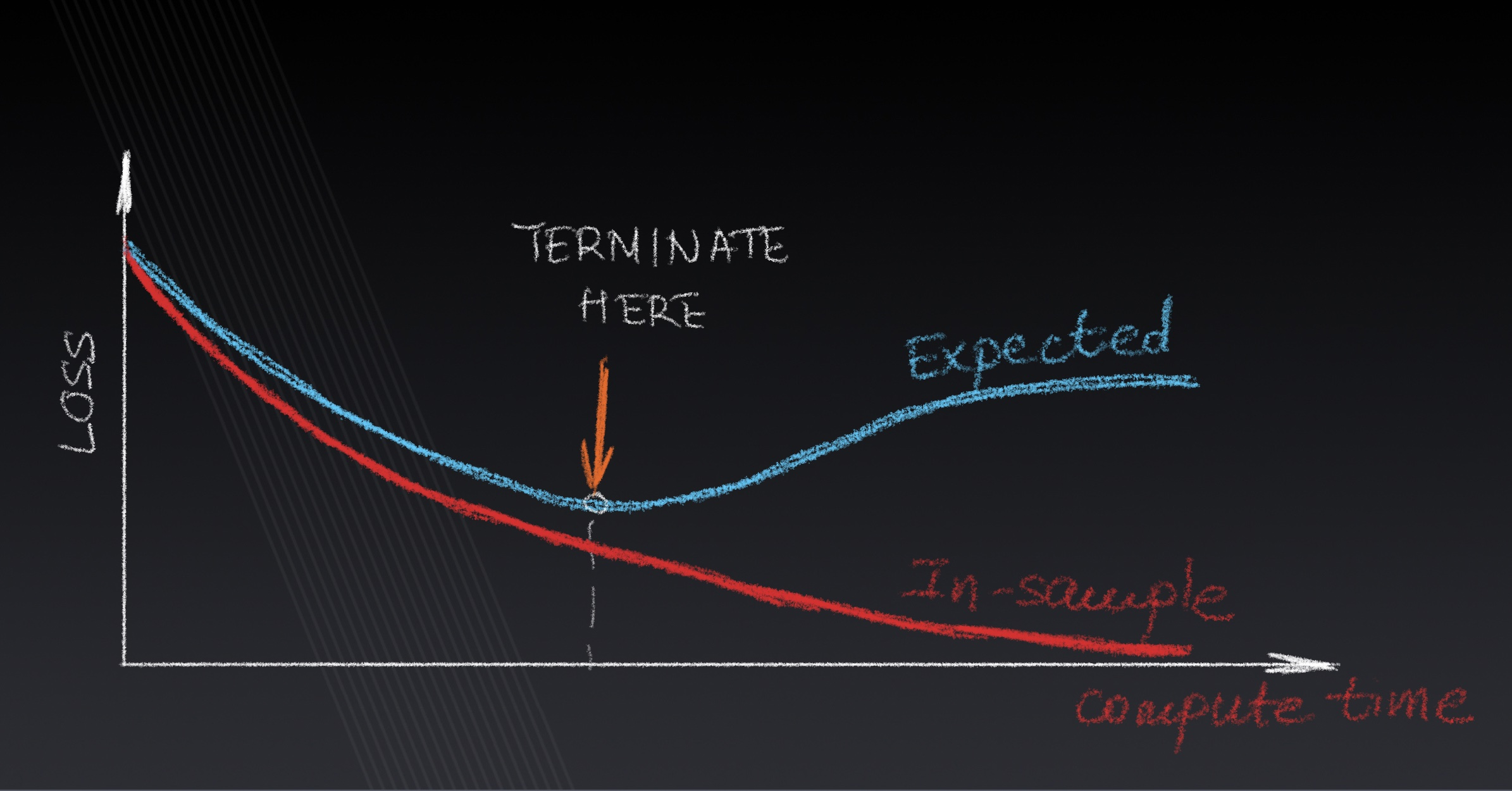## Lecture 4: Training Neural Networks

Approximation, estimation and optimization errors, regularization, loss surface curvature, descent-based optimization methods, second-order methods.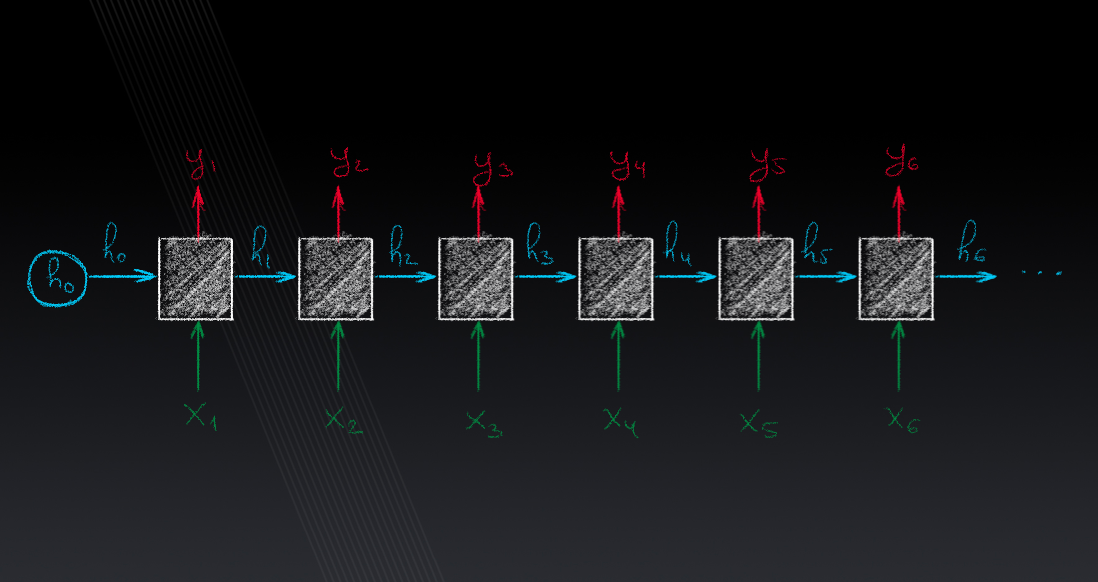## Lecture 5: Recurrent Neural Networks

RNN model, input-output sequences relationships, non-sequential input, layered RNN, backpropagation through time, word embeddings.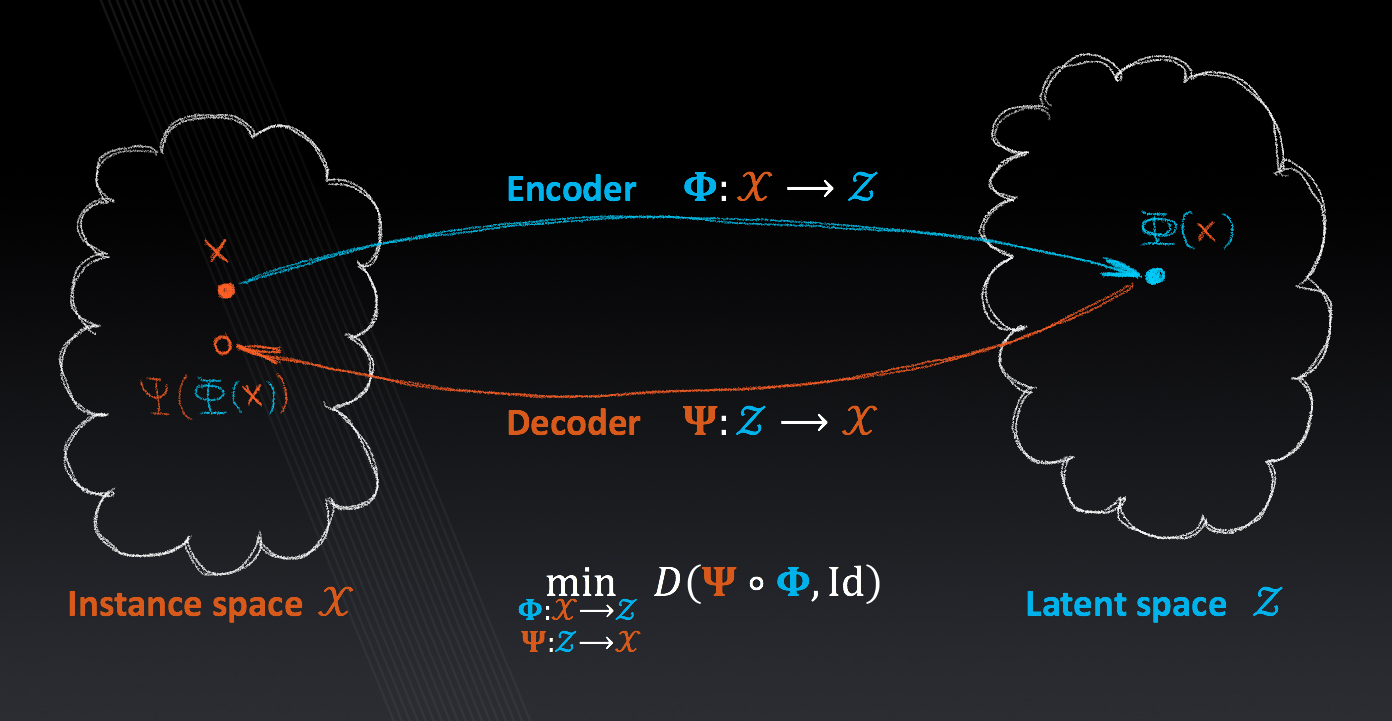## Lecture 6: Unsupervised Learning and Generative Models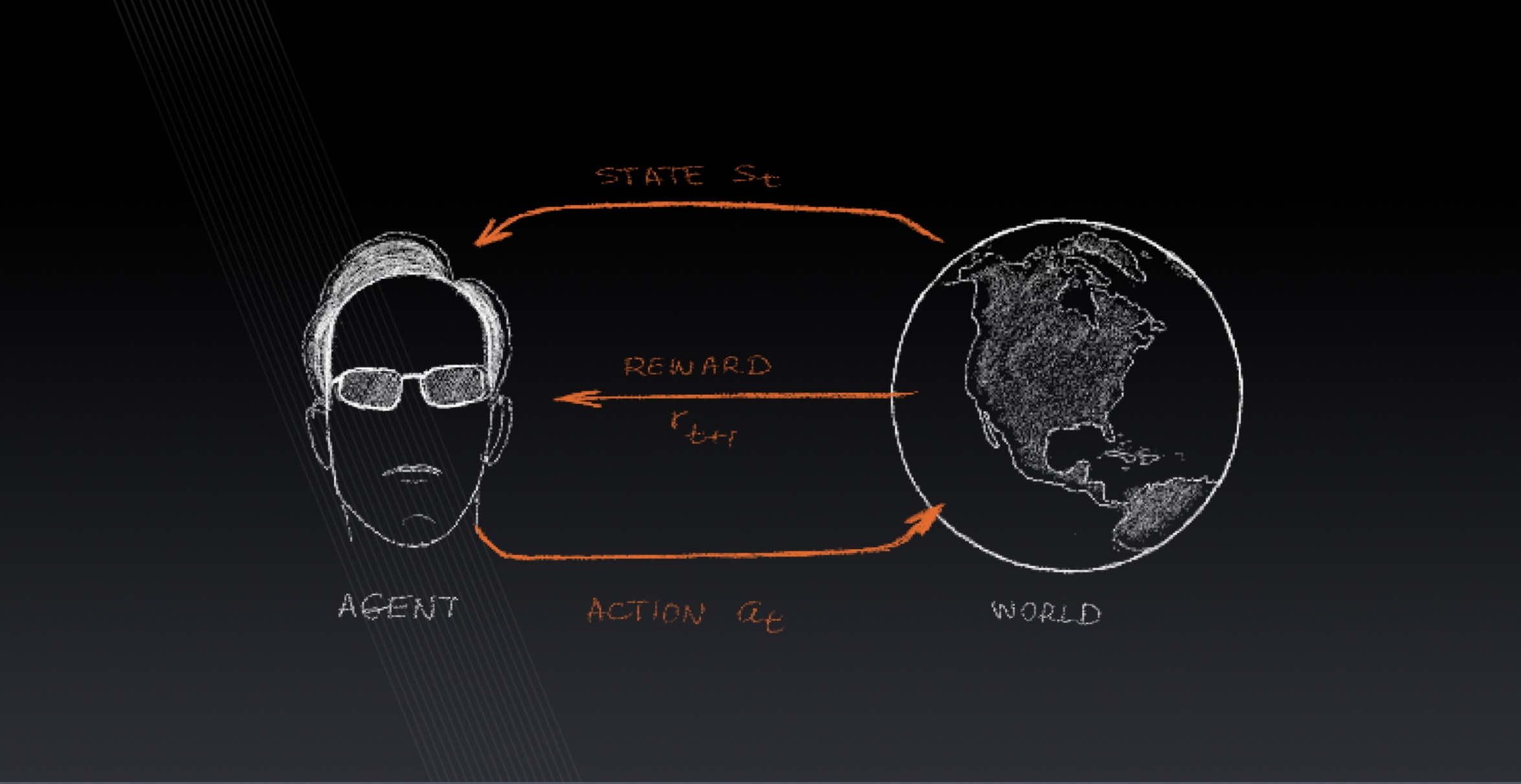## Lecture 7: Reinforcement Learning

Markov decision process, policies, rewards, value functions, the Bellman equation, q-learning, policy learning, actor-critic learning, AutoML.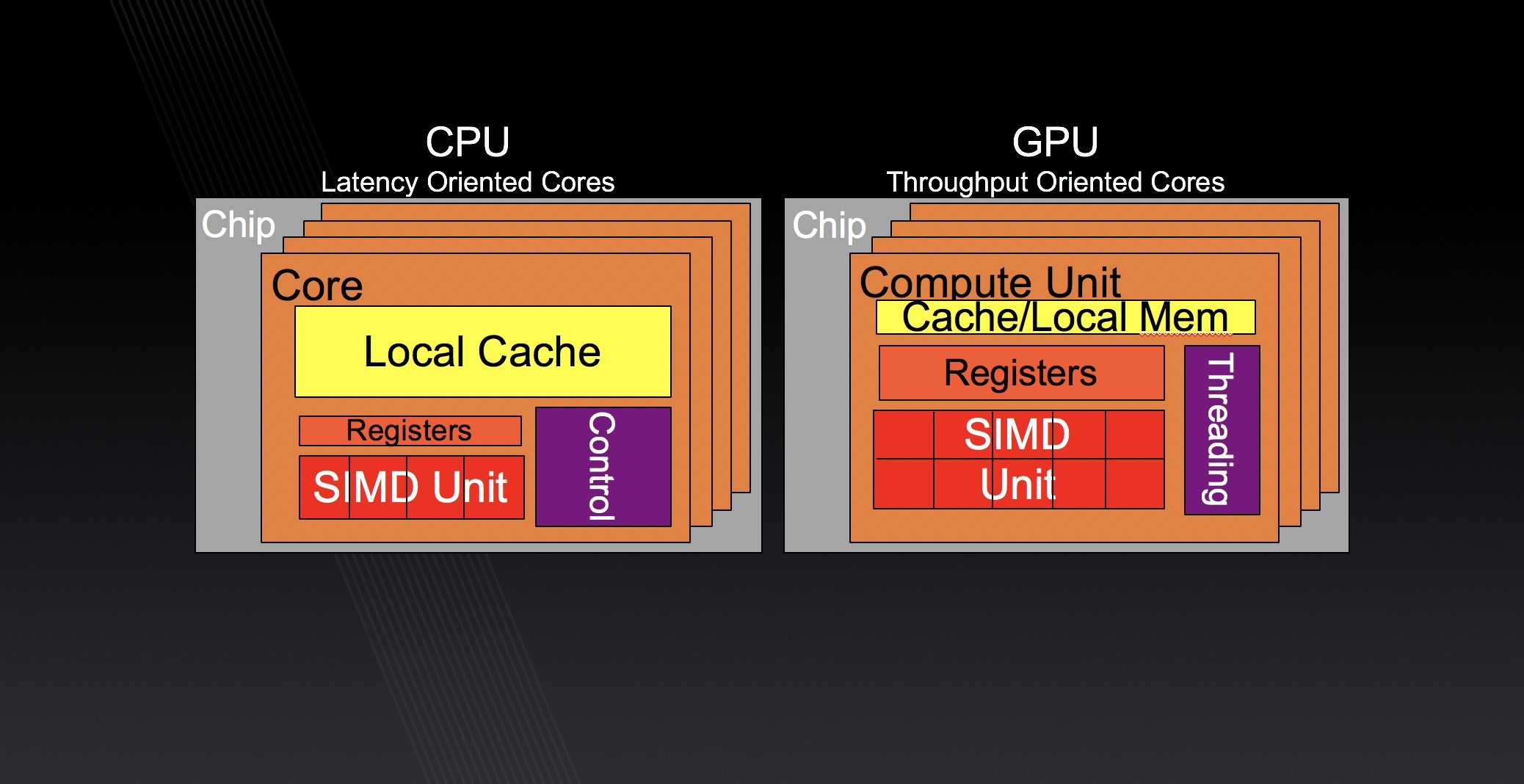## Lecture 8: Intro to Parallel Architectures

Parallelism and multithreading, execution of DL tasks, CPU vs. GPU architectures, CUDA programming model.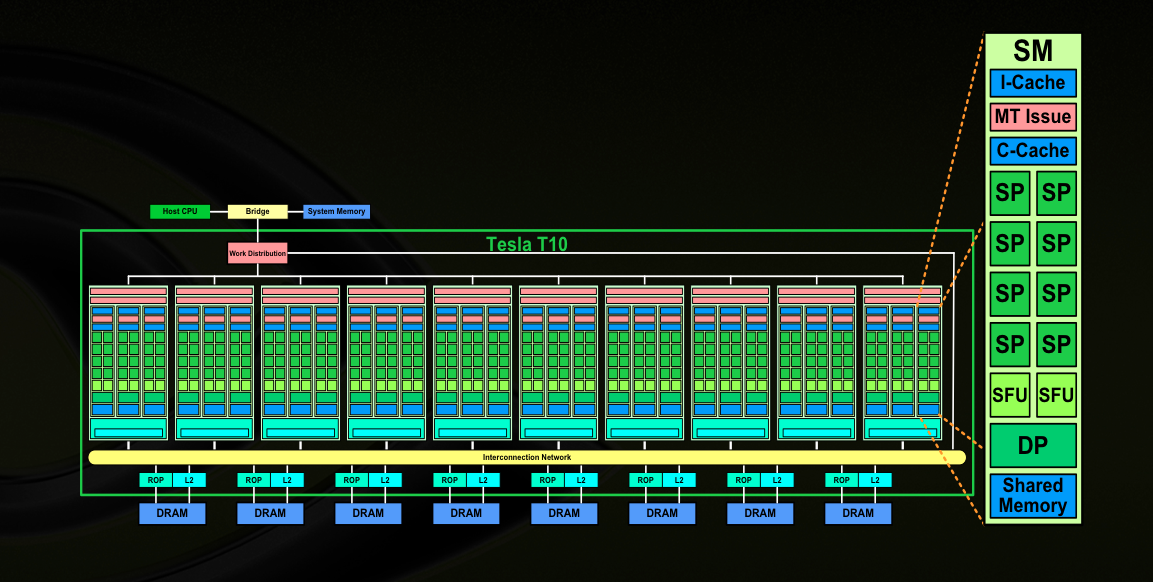## Lecture 9: Parallel Architectures for Training

NVidia GPU architectures, memory hierarchy, CUDA threads, unified memory, optimizations for CNNs, hardware architectures for training.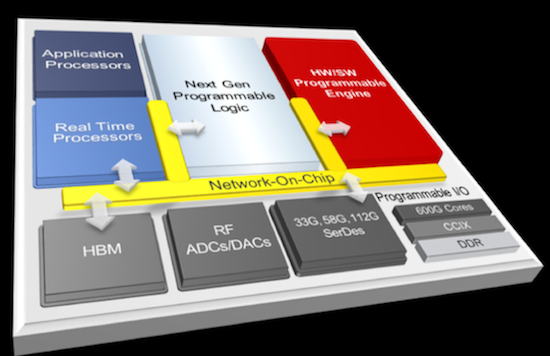## Lecture 10: Parallel Architectures for Inference

Challenges of inference, low-bit representations, pruning, GPU vs FPGA and ASIC, TPU architecture.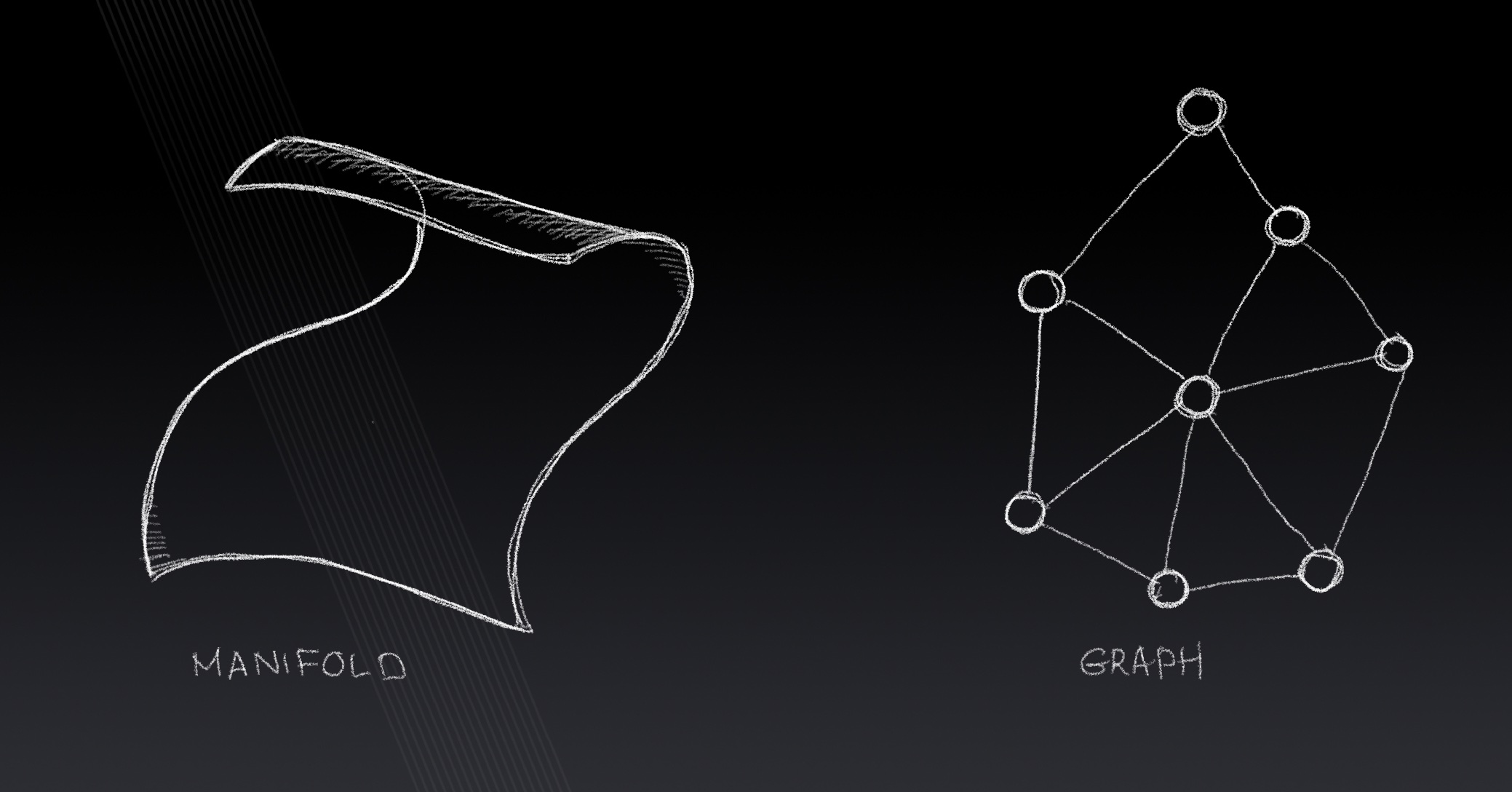## Lecture 11: Learning on Non-Euclidean Domains

Toeplitz operators, graphs, fields, gradients, divergence, Laplace-Beltrami operator, non-euclidean convolution, spectral and spatial CNN for graphs.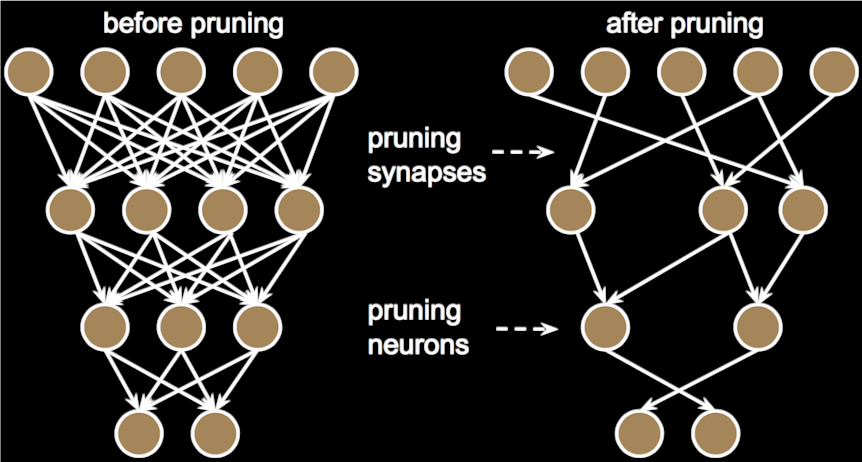## Lecture 12: Neural network compression

SVD for linear layers, flattened and grouped convolutions, pruning and retraining, network weights quantization.